# NCERT Solutions for Class 10 Maths Chapter 10 Circles

NCERT Solutions for Class 10 Maths Chapter 10 Circles - In our previous classes, you have learnt that a circle is a closed shape with a collection of points in a plane that are at a specific distance ( called the radius) from a fixed point (called center). Solutions of NCERT class 10 maths chapter 10 Circles is covering the in-depth explanations to questions related to a circle. You have also studied important terms related to the circle like segment, arc, sector, chord, etc. In this chapter, there are two exercises with 17 questions in them. CBSE NCERT solutions for class 10 maths chapter 10 Circles are solved by subject experts to help students in their preparation keeping step  by step marking in the mind.

This chapter introduces some complex and important terms like tangents, tangents to a circle, number of tangents from a point on a circle. In this chapter, we will study the different conditions that arise when a line and a circle are given in a plane. To solve these types of situations, we will learn the approach to apply the concept of the tangent to a circle in NCERT solutions for class 10 maths chapter 10 Circles. This chapter has fundamental concepts that are important for students in their future studies. Circles is a very interesting chapter due to the involvement of geometrical calculations and diagrams. These NCERT solutions can be used to study for competitive exams like JEE and NEET to build the basics.

## NCERT solutions for class 10 maths chapter 10 Circles Excercise: 10.1

The lines that intersect the circle exactly at one single point are called tangents. In a circle, there can be infinitely many tangents.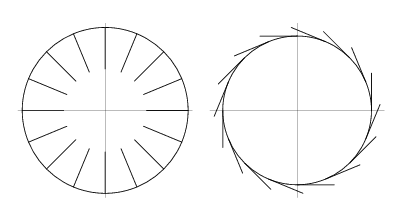(a) one
A tangent of a circle intersects the circle exactly in one single point.

(b) secant
It is a line that intersects the circle at two points.

(c) Two,
There can be only two parallel tangents to a circle.

(d) point of contact
The common point of a tangent and a circle.

(A) 12 cm

(B) 13 cm

(C) 8.5 cm

(D) cm.

The correct option is (d) =  cm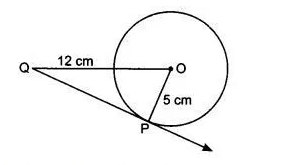It is given that the radius of the circle is 5 cm. OQ = 12 cm
According to question,
We know that
So, triangle OPQ is a right-angle triangle. By using Pythagoras theorem,

cm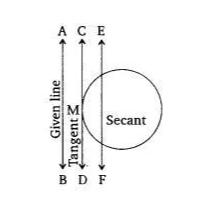AB is the given line and the line CD is the tangent to a circle at point M and parallels to the line AB. The line EF is a secant parallel to the AB

## NCERT solutions for class 10 maths chapter 10 Circles Excercise: 10.2

(A) 7 cm

(B) 12 cm

(C) 15 cm

(D) 24.5 cm

The correct option is (A) = 7 cmGiven that,
The length of the tangent (QT) is 24 cm and the length of OQ is 25 cm.
Suppose the length of the radius OT be  cm.
We know that  is a right angle triangle. So, by using Pythagoras theorem-

OT = 7 cm

## Circles Excercise: 10.2(A)

(B)

(C)

(D)

The correct option is (b)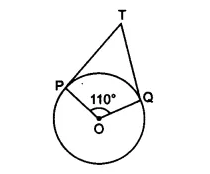In figure,
Since POQT is quadrilateral. Therefore the sum of the opposite angles are 180

(A) 50°

(B) 60°

(C) 70°

(D) 80°

The correct option is (A)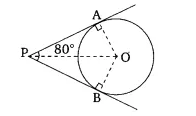It is given that,  tangent PA and PB from point P inclined at
In triangle OAP and OBP

OA =OB (radii of the circle)
PA = PB (tangents of the circle)

Therefore, by  SAS congruence

By CPCT,
Now, OPA = 80/4 = 40

In  PAO,

= 50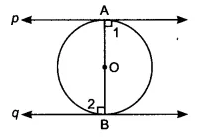Let line  and line  are two tangents of a circle and AB is the diameter of the circle.
OA and OB are perpendicular to the tangents  and   respectively.
therefore,

||  {  1  &  2 are alternate angles}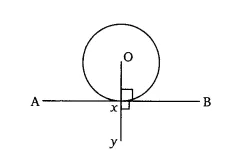In the above figure, the line AXB is the tangent to a circle with center O. Here, OX is the perpendicular to the tangent AXB () at point of contact X.
Therefore, we have,
BXO + YXB =

OXY is a collinear
OX is passing through the center of the circle.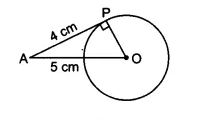Given that,
the length of the tangent from the point A (AP) is 4 cm and the length of OA is 5 cm.
Since APO = 900
Therefore,  APO is a right-angle triangle. By using Pythagoras theorem;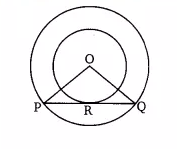In the above figure, Pq is the chord to the larger circle, which is also tangent to a smaller circle at the point of contact R.
We have,
radius of the larger circle OP = OQ = 5 cm
radius of the small circle (OR) = 3 cm

OR  PQ  [since PQ is tangent to a smaller circle]

According to question,

In OPR and OQR
PRO = QRO  {both }

OR = OR {common}

By RHS congruence OPR  OQR
So, by CPCT
PR = RQ
Now, In  OPR,
by using pythagoras theorem,

PR = 4 cm
Hence, PQ  = 2.PR =  8 cm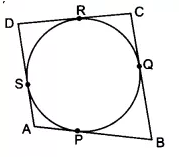To prove- AB + CD = AD + BC
Proof-
We have,
Since the length of the tangents drawn from an external point to a circle are equal
AP =AS .......(i)
BP = BQ.........(ii)
AS = AP...........(iii)
CR = CQ ...........(iv)

By adding all the equations, we get;

AP + BP +RD+ CR = AS +DS +BQ +CQ
(AP + BP) + (RD + CR) = (AS+DS)+(BQ + CQ)
AB + CD  = AD + BC

Hence proved.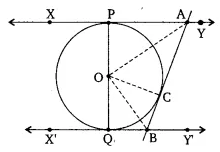To prove- AOB =
Proof-
In AOP and AOC,
OA =OA [Common]
AP =AC [tangents from external point A]
Therefore by SSS congruence,  AOP  AOC
and by CPCT,   PAO =  OAC
..................(i)

Similarly, from OBC and OBQ, we get;
QBC = 2.OBC.............(ii)

Adding eq (1) and eq (2)

PAC +  QBC = 180
2(OBC + OAC) = 180
(OBC + OAC) = 90

Now,  in  OAB,
Sum of interior angle is 180.
So, OBC + OAC + AOB = 180
AOB = 90
hence proved.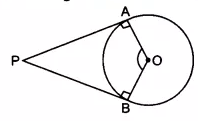To prove -
Proof-
We have, PA and PB are two tangents, B and A are the point of contacts of the tangent to a circle. And  ,  (since tangents and radius are perpendiculars)

According to question,
OAP + APB +PBO +BOA =
90 + APB + 90 +BOA = 360

Hence proved
.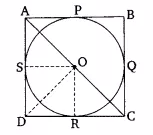To prove -  the parallelogram circumscribing a circle is a rhombus
Proof-
ABCD is a parallelogram that circumscribes a circle with center O.
P, Q, R, S are the points of contacts on sides AB, BC, CD, and DA  respectively
AB = CD .and AD = BC...........(i)
It is known that tangents drawn from an external point are equal in length.
RD = DS ...........(ii)
RC = QC...........(iii)
BP = BQ...........(iv)
AP = AS .............(v)
By adding eq (ii) to eq (v) we get;
(RD + RC) + (BP + AP) = (DS + AS) + (BQ + QC)
CD + AB = AD + BC
2AB = 2AD [from equation (i)]
Now, AB = AD and AB  = CD
AB = AD = CD = BC

Hence ABCD is a rhombus.Consider the above figure. Assume center O touches the sides AB and AC of the triangle at point E and F respectively.

Let the length of AE is x.

Now in ,

(tangents on the circle from point C)

(tangents on the circle from point B)

(tangents on the circle from point A)

Now AB = AE + EB

Now

Area of triangle

Now the area of

Area of

Area of

Now Area of the = Area of + Area of  + Area of

On squaring both the side, we get

Hence

AB = x + 8

=> AB = 7+8

=> AB = 15

AC = 6 + x

=> AC = 6 + 7

=> AC = 13

Answer-  AB = 15 and AC = 13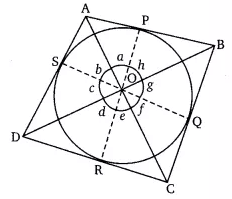Given- ABCD is a quadrilateral circumscribing a circle. P, Q, R, S are the point of contact on sides AB, BC, CD, and DA respectively.

To prove-

Proof -
Join OP, OQ, OR and OS
In triangle DOS and DOR,
OD =OD [common]
OS = OR [radii of same circle]
DR = DS [length of tangents drawn from an external point are equal ]
By SSS congruency, DOS  DOR,
and by CPCT, DOS = DOR
.............(i)

Similarily,
...............(2, 3, 4)

SImilarily,

Hence proved.

## NCERT solutions for class 10 maths chapter wise

 Chapter No. Chapter Name Chapter 1 CBSE NCERT solutions for class 10 maths chapter 1 Real Numbers Chapter 2 NCERT solutions for class 10 maths chapter 2 Polynomials Chapter 3 Solutions of NCERT class 10 maths chapter 3 Pair of Linear Equations in Two Variables Chapter 4 CBSE NCERT solutions for class 10 maths chapter 4 Quadratic Equations Chapter 5 NCERT solutions for class 10 chapter 5 Arithmetic Progressions Chapter 6 Solutions of NCERT class 10 maths chapter 6 Triangles Chapter 7 CBSE NCERT solutions for class 10 maths chapter 7 Coordinate Geometry Chapter 8 NCERT solutions for class 10 maths chapter 8 Introduction to Trigonometry Chapter 9 Solutions of NCERT class 10 maths chapter 9 Some Applications of Trigonometry Chapter 10 CBSE NCERT solutions class 10 maths chapter 10 Circles Chapter 11 NCERT solutions  for class 10 maths chapter 11 Constructions Chapter 12 Solutions of NCERT class 10 chapter maths chapter 12 Areas Related to Circles Chapter 13 CBSE NCERT solutions class 10 maths chapter 13 Surface Areas and Volumes Chapter 14 NCERT solutions for class 10 maths chapter 14 Statistics Chapter 15 Solutions of NCERT class 10 maths chapter 15 Probability

## How to use NCERT solutions for class 10 maths chapter 10 Circles?

• It is the chapter the logic as well as memory, both are tested equally.

• First, you should learn the theorems, terminologies, and concepts of circles.

• Once you have done the theorems, go through some examples of the NCERT textbook.

• When you have done the above-said points, then come to the practice exercises where your understanding of concepts will be tested.

• While doing the practice exercises, you can take help of NCERT solutions for class 10 maths chapter 10 circles.

• After doing all this, the last thing you should solve is past papers related to the particular chapter.

Keep working hard & happy learning!